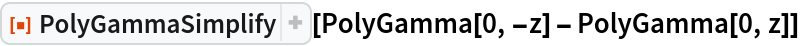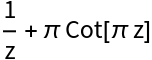Function Repository Resource:

# PolyGammaSimplify

Simplify polygamma functions in an expression

Contributed by: Wolfram Research
 ResourceFunction["PolyGammaSimplify"][expr] transforms polygamma functions in expr, trying to either decrease the number of polygamma functions or convert combinations of them into more elementary functions.

## Details and Options

A function that is essentially equivalent to ResourceFunction["PolyGammaSimplify"] is used inside FullSimplify and FunctionExpand.

## Examples

### Basic Examples (1)

Simplify an expression involving polygamma functions:

 In:=Out=## Requirements

Wolfram Language 11.3 (March 2018) or above

## Version History

• 1.0.0 – 06 March 2019